## Count on in tens (Christmas)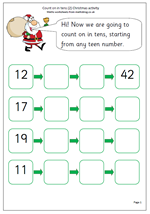We are rapidly approaching the Christmas season in schools, with plenty of work going on preparing concerts, nativities etc. but the maths work still needs to go on, so here are a couple of worksheets for Year 1 children with a Christmas feel to them.

They look at counting on in whole tens from any 2-digit number, with answers up to 100. It is important that children are confident and happy with counting in ones up to 100 before starting this and some children may still need a number square to help them with this. Counting on in tens also helps with the understanding of partitioning numbers into tens and units and it is important to ask children what is happening, both to the tens and the units.

Count on in tens (pg 2)

## New Maths Curriculum: Year 2 Multiplication and Division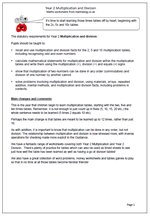Year 2 Multiplication and Division

It’s time to start learning those times tables off by heart; beginning with the 2x, 5x and 10x tables.
The statutory requirements for Year 2 Multiplication and division :

Pupils should be taught to:

• recall and use multiplication and division facts for the 2, 5 and 10 multiplication tables, including recognising odd and even numbers
• calculate mathematical statements for multiplication and division within the multiplication tables and write them using the multiplication (×), division (÷) and equals (=) signs
• show that multiplication of two numbers can be done in any order (commutative) and division of one number by another cannot
• solve problems involving multiplication and division, using materials, arrays, repeated addition, mental methods, and multiplication and division facts, including problems in contexts.

This is the year that children begin to learn multiplication tables, starting with the two, five and ten times tables. Remember, it is not enough to just count up in fives (5, 10, 15, 20 etc.) the whole sentence needs to be learned (5 times 2 equals 10 etc).
Perhaps the main change is that tables are meant to be learned up to 12 times, rather than just 10.

As with addition, it is important to know that multiplication can be done in any order, but not division. The relationship between multiplication and division is now stressed more, with inverse operations for checking made more explicit in the Guidance.

Year 2 multiplication and division statutory requirements

## New Maths Curriculum: Year 2 Addition and Subtraction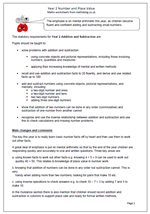Year 2 Addition and subtraction

The emphasis is on mental arithmetic this year, as children become fluent and confident adding and subtracting small numbers.

The statutory requirements for Year 2 Addition and Subtraction are:

Pupils should be taught to:

•    solve problems with addition and subtraction:

using concrete objects and pictorial representations, including those involving numbers, quantities and measures

applying their increasing knowledge of mental and written methods

•    recall and use addition and subtraction facts to 20 fluently, and derive and use related facts up to 100

•    add and subtract numbers using concrete objects, pictorial representations, and mentally, including:
a two-digit number and ones
a two-digit number and tens
two two-digit numbers

•    show that addition of two numbers can be done in any order (commutative) and subtraction of one number from another cannot

•    recognise and use the inverse relationship between addition and subtraction and use this to check calculations and missing number problems.

The key this year is to really learn basic number facts off by heart and then use them to work out other facts.
A great deal of emphasis is put on mental arithmetic so that by the end of the year children are responding quickly and accurately to oral and written questions. Three key areas are:

a. using known facts to work out other facts e.g. knowing 4 + 5 = 9 can be used to work out
quickly 40 + 50. This relates to knowledge of place value in number work.

b. knowing that addition of numbers can be done in any order but subtraction cannot. This is very
handy when adding more than two numbers; looking for pairs that make 10 etc.

c. using inverse operations to check answers e.g. to check 10 – 7 = 3 by adding 7 and 3 to make 10.

In the Guidance section there is also mention that children should record addition and subtraction in columns to support place vale and ready for formal written methods.

New Maths Programme of Study: Year 2 Addition and Subtraction

## New Maths Curriculum: Year 2 Number and Place Value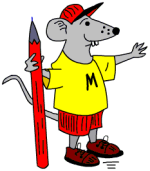There are some tough challenges in the new Year 2 Programme of Study. Let’s begin by looking at ‘Number and place value’.
The statutory requirements for Year 2 Number and Place Value are:

Number and place value

Pupils should be taught to:

•    count in steps of 2, 3, and 5 from 0, and in tens from any number, forward or backward

•    recognise the place value of each digit in a two-digit number (tens, ones)

•    identify, represent and estimate numbers using different representations, including the number line

•    compare and order numbers from 0 up to 100; use <, > and = signs

•    read and write numbers to at least 100 in numerals and in words

•    use place value and number facts to solve problems.

Main changes
Most of this looks very similar to the old curriculum. The main difference is the introduction of the < and > signs. Remembering which is which could prove very tricky.
Writing numbers to at least 100 in words is a real challenge which quite a few children will not be able to manage by the end of the year.
A key idea in this category is partitioning numbers in different ways. For example:
24 can be split up or partitioned into 20 + 4 or 10 + 14.
This is important to understand as it helps with written addition and subtraction in later years. Also it is important to begin to understand zero as a place holder; i.e. the 1 in 100 has a value ten times the 1 in 10, with the zeros being used as place holders.
Counting in multiples of 3 is a new target, introduced to help later with understanding the concept of a third.
Also note that this new programme will not become a statutory requirement until 2015.

Year 2 Number and Place Value

## New Maths Curriculum: Year 1 Geometry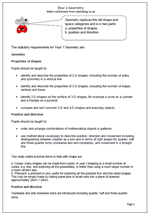Geometry replaces the old shape and space categories and is in two parts:

a. properties of shapes
b. position and direction.

The statutory requirements for Year 1 Geometry are:

Properties of shapes

Pupils should be taught to:

•    identify and describe the properties of 2-D shapes, including the number of sides and symmetry in a vertical line

•    identify and describe the properties of 3-D shapes, including the number of edges, vertices and faces

•    identify 2-D shapes on the surface of 3-D shapes, for example a circle on a cylinder and a triangle on a pyramid

•    compare and sort common 2-D and 3-D shapes and everyday objects.

Position and direction

Pupils should be taught to:

•    order and arrange combinations of mathematical objects in patterns

•    use mathematical vocabulary to describe position, direction and movement including distinguishing between rotation as a turn and in terms of right angles for quarter, half and three-quarter turns (clockwise and anti-clockwise), and movement in a straight line.

Two really useful practical items to help with shape are:

a. Cubes: many shapes can be made from cubes. In year 2 keeping to a small number of cubes, e.g. four, and exploring all the possibilities, is better than using a much larger number in a more random way.
b. Pinboard: a pinboard is very useful for exploring all the possible four and five sided shapes. This may be simply made by nailing panel pins or small nails into a piece of plywood approximately 24cm × 24cm.

Position and direction
Clockwise and anti-clockwise turns are introduced including quarter, half and three-quarter turns.

Year 1 Geometry: statutory requirements

## New Maths Curriculum: Year 1 Measurement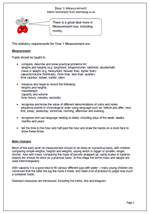Here are the statutory requirements for Measurement in Year 1. There is a great deal more in Measurement now, including money and time.

Measurement

Pupils should be taught to:

•    compare, describe and solve practical problems for:
lengths and heights (e.g. long/short, longer/shorter, tall/short, double/half)
mass or weight (e.g. heavy/light, heavier than, lighter than)
capacity/volume (full/empty, more than, less than, quarter)
time (quicker, slower, earlier, later)

•    measure and begin to record the following:
lengths and heights
mass/weight
capacity and volume
time (hours, minutes, seconds)

•    recognise and know the value of different denominations of coins and notes
sequence events in chronological order using language such as: before and after, next,
first, today, yesterday, tomorrow, morning, afternoon and evening

•    recognise and use language relating to dates, including days of the week, weeks, months and years

•    tell the time to the hour and half past the hour and draw the hands on a clock face to show these times.

Main changes

Much of the early work on measurement should to be done on a practical basis, with children comparing simple lengths, heights and weights, saying which is bigger or smaller, longer, shorter. Also with mass, comparing the mass of parcels wrapped up, using scales to balance objects etc should be done on a practical basis. At this stage the terms mass and weight are used interchangeably.

With capacity it is a good idea to fill various different jugs with water – many young children are convinced that the taller the jug the more it holds, and need a lot of practice to judge how much a container holds.

Standard measures are introduced, including the metre, litre and kilogram.

Year 1 Measurement statutory requirements

## New Maths Curriculum: Year 1 Fractions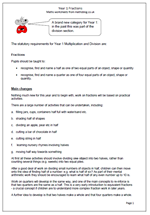Fractions is a brand new category for Year 1: in the past this was part of the division section.

The statutory requirements for Year 1 Multiplication and Division are:

Fractions

Pupils should be taught to:

•    recognise, find and name a half as one of two equal parts of an object, shape or quantity

•    recognise, find and name a quarter as one of four equal parts of an object, shape or quantity.

Main changes

Nothing much new for this year and to begin with, work on fractions will be based on practical activities.

There are a large number of activities that can be undertaken, including:

a.    filling jars, cups, containers half full with water/sand etc.

c.    dividing an apple, pear etc in half

d.    cutting a bar of chocolate in half

e.    cutting string in half

f.     learning nursery rhymes involving halves

g.    moving half way towards something

At first all these activities should involve dividing one object into two halves, rather than counting several things (e.g. sweets) into two equal piles.

After a good deal of work on dividing small numbers of objects in half, children can then move onto the idea of finding half of a number: e.g. what is half of six? As part of their mental arithmetic work they should be encouraged to learn what half of any even number up to 10 is.

Work on quarters will develop in the same way, and one of the main concepts to re-inforce is that two quarters are the same as a half. This is a very early introduction to equivalent fractions – a crucial concept if children are to understand more complex fraction work in later years.

A further idea to develop is that two halves make a whole and that four quarters make a whole.

Year 1 Fractions: statutory requirements

## New Maths Curriculum: Year 1 Multiplication and Division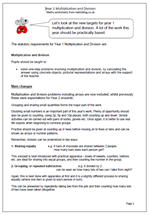Let’s look at the new targets for year 1 multiplication and division. A lot of the work this year should be practically based.

The statutory requirements for Year 1 Multiplication and Division are:

Multiplication and division

Pupils should be taught to:

•    solve one-step problems involving multiplication and division, by calculating the answer using concrete objects, pictorial representations and arrays with the support of the teacher.

Main changes

Multiplication and division problems including arrays are now included, whilst previously these were expectations for Year 2 onwards.

Grouping and sharing small quantities forms the major part of the work.

Doubling small numbers is an important part of this year’s work. Plenty of opportunity should also be given to counting, using 2p, 5p and 10p pieces, both counting up and down. Similar activities can be carried out with pairs of socks, gloves etc. Once again, it is better to use real life objects when beginning to combine groups.

Practice should be given at counting up in twos before moving on to fives or tens and can be shown as arrays or number patterns.
Remember, division can be understood in two ways:

1. Sharing equally               e.g. 6 bars of chocolate are shared between 2 people.
How many bars does each person get?

This concept is best introduced with practical apparatus – bowls of sweets, counters, buttons etc. are ideal for sharing into equal groups, and then counting the number in the group.

2. Grouping, or repeated subtraction                     e.g. 8 divided by 2
can be seen as how many lots of two can I take from eight?

Again, this is best done with apparatus at first and it is a slightly different process to sharing equally (where one item is given to each person in turn).

This can be answered by repeatedly taking two from the pile and then counting how many lots of two have been taken altogether.

Multiplication and division in Year 1

## New Maths Curriculum: Year 1 Addition and Subtraction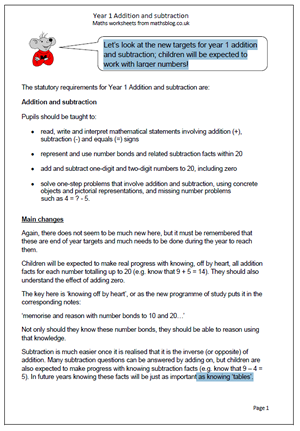Let’s look at the new targets for year 1 addition and subtraction; children will be expected to work with larger numbers!

Pupils should be taught to:

•    read, write and interpret mathematical statements involving addition (+), subtraction (-) and equals (=) signs

•    represent and use number bonds and related subtraction facts within 20

•    add and subtract one-digit and two-digit numbers to 20, including zero

•    solve one-step problems that involve addition and subtraction, using concrete objects and pictorial representations, and missing number problems
such as 4 = ? – 5.

Again, there does not seem to be much new here, but it must be remembered that these are end of year targets and much needs to be done during the year to reach them.
Children will be expected to make real progress with knowing, off by heart, all addition facts for each number totalling up to 20 (e.g. know that 9 + 5 = 14). They should also understand the effect of adding zero.
The key here is ‘knowing off by heart’, or as the new programme of study puts it in the corresponding notes:
‘memorise and reason with number bonds to 10 and 20…’
Not only should they know these number bonds, they should be able to reason using that knowledge.
Subtraction is much easier once it is realised that it is the inverse (or opposite) of addition. Many subtraction questions can be answered by adding on, but children are also expected to make progress with knowing subtraction facts (e.g. know that 9 – 4 = 5). In future years knowing these facts will be just as important as knowing ‘tables’.

New programme of Study: Year 1 Addition and Subtractionddition and subtraction

## New Maths Curriculum: Year 1 Number and Place ValueIt’s a whole new set of targets for Year 1 from 2014. Let’s begin by having a look at Number and Place Value.

The statutory requirements for Year 1 Number and Place Value are:

Number and place value

Pupils should be taught to:

•    count to and across 100, forwards and backwards, beginning with 0 or 1, or from any given number

•    count, read and write numbers to 100 in numerals; count in multiples of twos, fives and tens

•    given a number, identify one more and one less

•    identify and represent numbers using objects and pictorial representations including the number line, and use the language of: equal to, more than, less than (fewer), most, least

•    read and write numbers from 1 to 20 in numerals and words.

Main changes

The new maths programme of study is much shorter than previous models with much broader statements. This makes it tricky to know what has been left out. However, the main aim is to raise standards by raising targets to be achieved. In Year 1 the main changes are:

1. Counting, reading and writing numbers to 100 rather than just up to 20. This will prove to be very difficult for many children and will need a great deal of practice.
2. Perhaps even harder is to count in twos, fives and tens from different multiples.

New Statutory Requirements 2014:  Number and Place Value